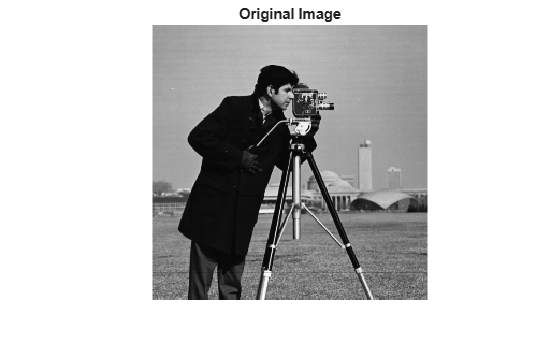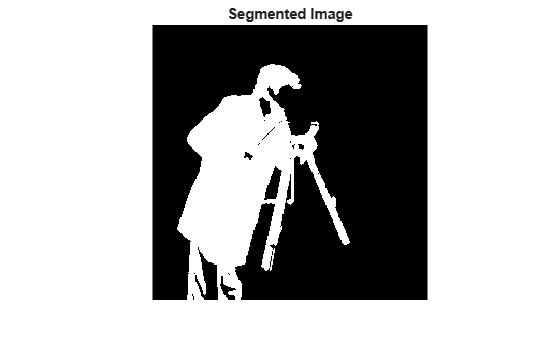# graydiffweight

Calculate weights for image pixels based on grayscale intensity difference

## Syntax

``W = graydiffweight(I,refGrayVal)``
``W = graydiffweight(I,mask)``
``W = graydiffweight(I,C,R)``
``W = graydiffweight(V,C,R,P)``
``W = graydiffweight(___, Name,Value)``

## Description

````W = graydiffweight(I,refGrayVal)` computes the pixel weight for each pixel in the grayscale image `I`. The weight is the absolute value of the difference between the intensity of the pixel and the reference grayscale intensity specified by the scalar `refGrayVal`. Pick a reference grayscale intensity value that is representative of the object you want to segment. The weights are returned in the array `W`, which is the same size as input image `I`. The weight of a pixel is inversely related to the absolute value of the grayscale intensity difference at the pixel location. If the difference is small (intensity value close to `refGrayVal`), the weight value is large. If the difference is large (intensity value very different from `refGrayVal`), the weight value is small.```
````W = graydiffweight(I,mask)` computes the pixel weights, where the reference grayscale intensity value is the average of the intensity values of all the pixels in `I` that are marked as logical `true` in `mask`. Using the average of several pixels to calculate the reference grayscale intensity value can be more effective than using a single reference intensity value, as in the previous syntax.```
````W = graydiffweight(I,C,R)` computes the pixel weights, where the reference grayscale intensity value is the average of the intensity values of the pixel locations specified by the vectors `C` and `R`. `C` and `R` contain the column and row indices of the pixel locations that must be valid pixel indices in `I`.```
````W = graydiffweight(V,C,R,P)` computes the weights for each voxel in the volume `V`, specified by the vectors `C`, `R`, and `P`. `C`, `R`, and `P` contain the column, row, and plane indices of the voxel locations that must be valid voxel indices in `V`.```

example

````W = graydiffweight(___, Name,Value)` returns the weight array `W` using name-value pairs to control aspects of weight computation.```

## Examples

collapse all

This example segments an object in an image using Fast Marching Method using grayscale intensity difference weights calculated from the intensity values at the seed locations.

```I = imread('cameraman.tif'); imshow(I) title('Original Image')```Specify row and column index of pixels for use a reference grayscale intensity value.

```seedpointR = 159; seedpointC = 67;```

Calculate the grayscale intensity difference weight array for the image and display it. The example does log-scaling of `W` for better visualization.

```W = graydiffweight(I, seedpointC, seedpointR,'GrayDifferenceCutoff',25); figure, imshow(log(W),[])```Segment the image using the grayscale intensity difference weight array. Specify the same seed point vectors you used to create the weight array.

```thresh = 0.01; BW = imsegfmm(W, seedpointC, seedpointR, thresh); figure, imshow(BW) title('Segmented Image')```## Input Arguments

collapse all

Grayscale image, specified as a 2-D numeric matrix.

Data Types: `single` | `double` | `int8` | `uint8` | `int16` | `uint16` | `int32` | `uint32`

Grayscale volume, specified as a 3-D numeric array.

Data Types: `single` | `double` | `int8` | `uint8` | `int16` | `uint16` | `int32` | `uint32`

Reference grayscale intensity value, specified as a scalar.

Data Types: `double`

Reference grayscale intensity mask, specified as a logical array of the same size as `I`.

Data Types: `logical`

Column index of reference pixel (or voxel), specified as a numeric (integer-valued) vector.

Data Types: `double`

Row index of reference pixel (or voxel), specified as a numeric (integer-valued) vector.

Data Types: `double`

Plane index of reference voxel, specified as a numeric (integer-valued) vector.

Data Types: `double`

### Name-Value Pair Arguments

Specify optional comma-separated pairs of `Name,Value` arguments. `Name` is the argument name and `Value` is the corresponding value. `Name` must appear inside quotes. You can specify several name and value pair arguments in any order as `Name1,Value1,...,NameN,ValueN`.

Example: `W = graydiffweight(I, seedpointC, seedpointR,'GrayDifferenceCutoff',25);`

Output weight roll-off factor, specified as the comma-separated pair consisting of `'RolloffFactor'` and a positive scalar of class `double`. Controls how fast the output weight falls as the function of the absolute difference between an intensity value and the reference grayscale intensity. When viewed as a 2-D plot, pixel intensity values can vary gradually at the edges of regions, creating a gentle slope. In your segmented image, you might want the edge to be more well-defined. Using the roll-off factor, you control the slope of the weight value curve at points where intensity values start to change. If you specify a high value, the output weight values fall off sharply around the regions of change intensity. If you specify a low value, the output weight has a more gradual fall-off around the regions of changing intensity. The suggested range for this parameter is `[0.5 4]`.

Data Types: `double`

Threshold for absolute grayscale intensity difference values, specified as the comma-separated pair consisting of `'GrayDifferenceCutoff'` and a nonnegative scalar of class `double`. When you put a threshold on intensity difference values, you strongly suppress output weight values greater than the cutoff value. `graydiffweight` assigns these pixels the smallest weight value. When the output weight array `W` is used for Fast Marching Method based segmentation (as input to `imsegfmm`), this parameter can be useful in improving the accuracy of the segmentation output. Default value of this parameter is `Inf`, which means that there is no hard cutoff.

Data Types: `double`

## Output Arguments

collapse all

Weight array, specified as numeric array of the same size as the input image `I` or volume `V`. `W` is of class `double`, unless the input image or volume is of class `single`, in which case `W` is of class `single`.